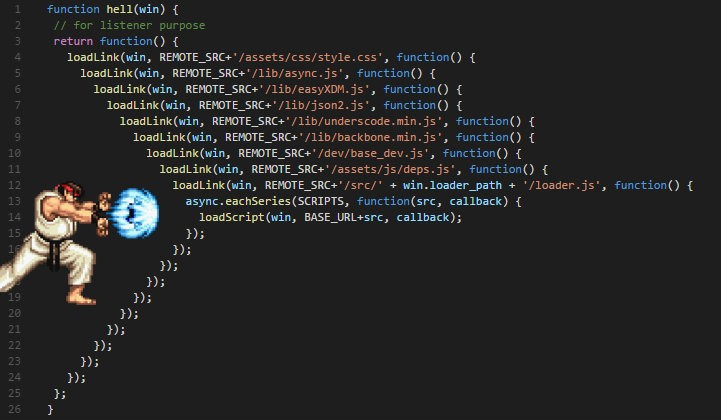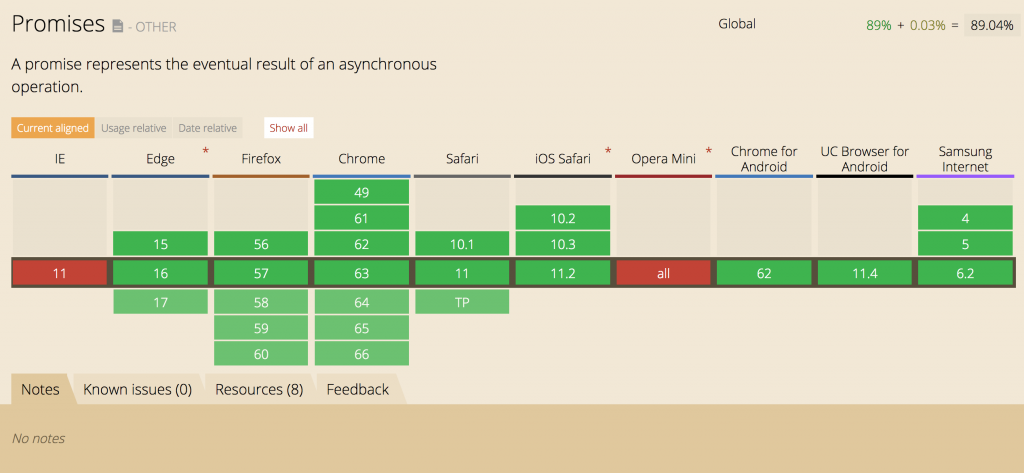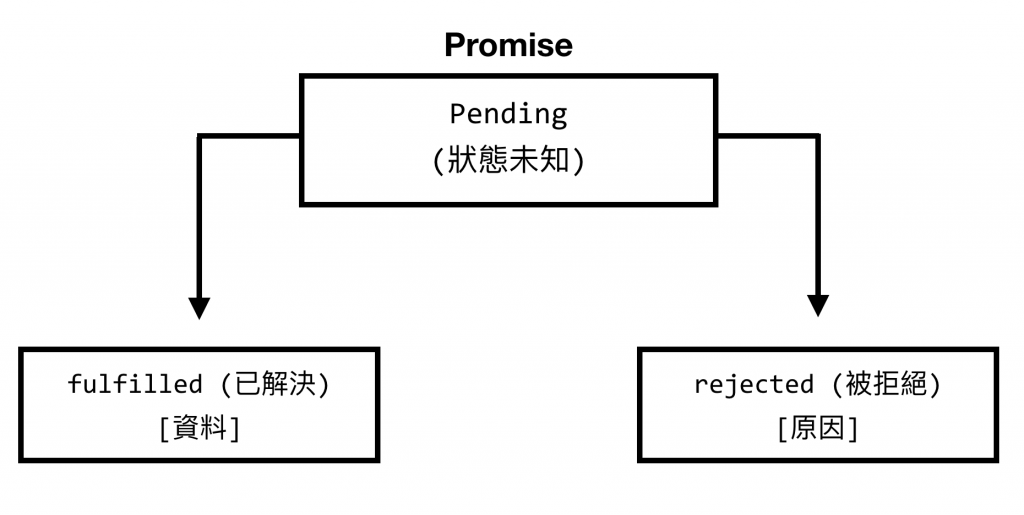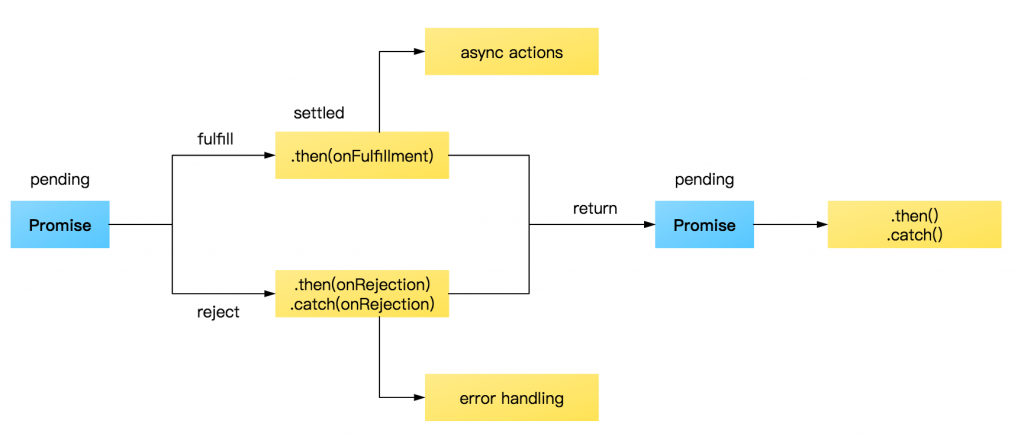#2018 iT 邦幫忙鐵人賽DAY 26
7
Modern Web

## 解釋「同步」與「非同步」.
.
.

JavaScript 是一個「非同步」的語言，所以當我們執行這段程式時， `for` 迴圈並不會等待 `window.setTimeout` 結束後才繼續，而是在執行階段就一口氣跑完。## 再見 Callback Hell

``````var funcA = function(){
var i = Math.random() + 1;

window.setTimeout(function(){
console.log('function A');
}, i * 1000);
};

var funcB = function(){
var i = Math.random() + 1;

window.setTimeout(function(){
console.log('function B');
}, i * 1000);
};

funcA();
funcB();
``````

``````var funcA = function(callback){
var i = Math.random() + 1;

window.setTimeout(function(){
console.log('function A');

// 如果 callback 是個函式就呼叫它
if( typeof callback === 'function' ){
callback();
}

}, i * 1000);
};

var funcB = function(){
var i = Math.random() + 1;

window.setTimeout(function(){
console.log('function B');
}, i * 1000);
};

// 為了確保先執行 funcA 再執行 funcB, 呼叫 funcA() 的時候，將 funcB 作為參數帶入
funcA( funcB );
``````*「波動拳」(a.k.a. "Callback Hell") *

``````var result = [];
var step = 3;

// 假設 funcA、funcB、funcC 分別代表「切青菜、切番茄、擺盤」
function funcA(){
window.setTimeout(function(){
result.push('A');
console.log('A');

if( result.length === step ){
funcD();
}

}, (Math.random() + 1) * 1000);
}

function funcB(){
window.setTimeout(function(){
result.push('B');
console.log('B');

if( result.length === step ){
funcD();
}
}, (Math.random() + 1) * 1000);
}

function funcC(){
window.setTimeout(function(){
result.push('C');
console.log('C');

if( result.length === step ){
funcD();
}
}, (Math.random() + 1) * 1000);
}

function funcD(){
console.log('上菜！');
result = [];
}

funcA();
funcB();
funcC();
``````

``````function serials(tasks, callback) {
var result = [];

// 檢查的邏輯寫在這裡
function check(r) {
result.push(r);
if( result.length === step ){
callback();
}
}

f(check);
});
}
``````

``````function funcA(check){
window.setTimeout(function(){
console.log('A');
check('A');
}, (Math.random() + 1) * 1000);
}

function funcB(check){
window.setTimeout(function(){
console.log('B');
check('B');
}, (Math.random() + 1) * 1000);
}

function funcC(check){
window.setTimeout(function(){
console.log('C');
check('C');
}, (Math.random() + 1) * 1000);
}

function funcD(){
console.log('上菜！');
}
``````

``````serials([funcA, funcB, funcC], funcD);
``````

## Promise 物件https://caniuse.com/#feat=promises

``````const myFirstPromise = new Promise((resolve, reject) => {
resolve(someValue);         // 完成
reject("failure reason");   // 拒絕
});
``````

``````function myAsyncFunction(url) {
return new Promise((resolve, reject) => {
// resolve() or reject()
});
};
``````

`Promise` 被完成的時候，我們就可以呼叫 `resolve()`，然後將取得的資料傳遞出去。 或是說想要拒絕 `Promise`當個完全沒有信用的人， 那麼就呼叫 `reject()` 來拒絕。

• pending: 初始狀態，不是 fulfilled 或 rejected。
• fulfilled: 表示操作成功地完成。
• rejected: 表示操作失敗。``````function funcA(){
return new Promise(function(resolve, reject){
window.setTimeout(function(){
console.log('A');
resolve('A');
}, (Math.random() + 1) * 1000);
});
}

function funcB(){
return new Promise(function(resolve, reject){
window.setTimeout(function(){
console.log('B');
resolve('B');
}, (Math.random() + 1) * 1000);
});
}

function funcC(){
return new Promise(function(resolve, reject){
window.setTimeout(function(){
console.log('C');
resolve('C');
}, (Math.random() + 1) * 1000);
});
}
``````

``````funcA().then(funcB).then(funcC);
``````

``````// funcA, funcB, funcC 的先後順序不重要
// 直到這三個函式都回覆 resolve 或是「其中一個」 reject 才會繼續後續的行為

Promise.all([funcA(), funcB(), funcC()])
.then(function(){ console.log('上菜'); });
``````

• [註1] : Overcooked 在 PS4 / switch 平台上都有，是個極歡樂的友情破壞遊戲，但只有一個人玩的話極度不推薦 QQ。
• [註2] : 鄉民說的其實是「不是被打一巴掌，就是被關一輩子」切勿以身試法

### 5 則留言

1

1

funcA().then(funcB).then(funcC);

funcA().then(funcB()).then(funcC());

Kuro Hsu iT邦新手 4 級 ‧ 2019-05-23 13:55:05 檢舉

Amigo iT邦新手 5 級 ‧ 2019-05-23 17:44:48 檢舉

function funcX(x){
return new Promise(function(resolve, reject){
window.setTimeout(function(){
console.log(x);
resolve(x);
}, (Math.random() + 1) * 1000);
});
}

Kuro Hsu iT邦新手 4 級 ‧ 2019-05-23 18:24:43 檢舉
``````function funcA(val){
return new Promise(function(resolve, reject){
window.setTimeout(function(){
console.log('A');
resolve(val);
}, (Math.random() + 1) * 1000);
});
}

function funcB(val){
return new Promise(function(resolve, reject){
window.setTimeout(function(){
console.log('B', val);
resolve(val);
}, (Math.random() + 1) * 1000);
});
}

function funcC(val){
return new Promise(function(resolve, reject){
window.setTimeout(function(){
console.log('C', val);
resolve(val);
}, (Math.random() + 1) * 1000);
});
}

funcA(123).then(funcB).then(funcC);
``````

Amigo iT邦新手 5 級 ‧ 2019-05-24 11:12:16 檢舉

funcX(3).then(()=>funcX(1)).then(()=>funcX(2))

0

• 更改 a執行時間設為較長、b較短
``````var funcA = function(callback){
window.setTimeout(function(){
console.log('function A');
if( typeof callback === 'function' ){ callback(); }
}, 3000);
};

var funcB = function(){
window.setTimeout(function(){
console.log('function B');
}, 1000);
};

// 為了確保先執行 funcA 再執行 funcB, 呼叫 funcA() 的時候，將 funcB 作為參數帶入
funcA( funcB );
// B先執行 才執行A ????
funcA( funcB() )
``````
Kuro Hsu iT邦新手 4 級 ‧ 2019-05-30 10:08:29 檢舉

RocMark iT邦新手 5 級 ‧ 2019-05-30 16:36:47 檢舉

0

1.serials([funcA, funcB, funcC], funcD); 傳給function serials()

2.function serials() 執行
f(check); //---Q1
});

==>funcA( check ) 嗎?

3.接下來 他去執行function funcA(check){ //----Q5 嗎?

if( result.length === step ){
callback(); //---Q2
}

``````function serials(tasks, callback) {
var result = [];

// 檢查的邏輯寫在這裡
function check(r) {  //---Q3
result.push(r);
if( result.length === step ){
callback();  //---Q2
}
}

f(check);  //---Q1
});
}

function funcA(check){  //----Q5
window.setTimeout(function(){
console.log('A');
check('A');  ////---Q4
}, (Math.random() + 1) * 1000);
}

function funcB(check){
window.setTimeout(function(){
console.log('B');
check('B');
}, (Math.random() + 1) * 1000);
}

function funcC(check){
window.setTimeout(function(){
console.log('C');
check('C');
}, (Math.random() + 1) * 1000);
}

function funcD(){
console.log('上菜！');
}

serials([funcA, funcB, funcC], funcD);
``````
0

Kuro Hsu iT邦新手 4 級 ‧ 2019-09-20 10:20:52 檢舉

Amigo iT邦新手 5 級 ‧ 2019-09-20 11:08:02 檢舉